Скачать презентацию Group Analysis File Group Ana pdf Gang Chen

f77b001467c89bac390a52702619496e.ppt

• Количество слайдов: 52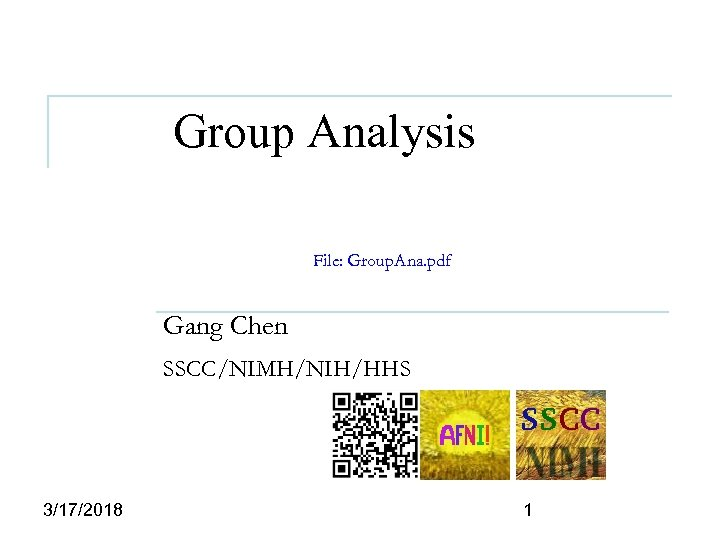Group Analysis File: Group. Ana. pdf Gang Chen SSCC/NIMH/NIH/HHS 3/17/2018 1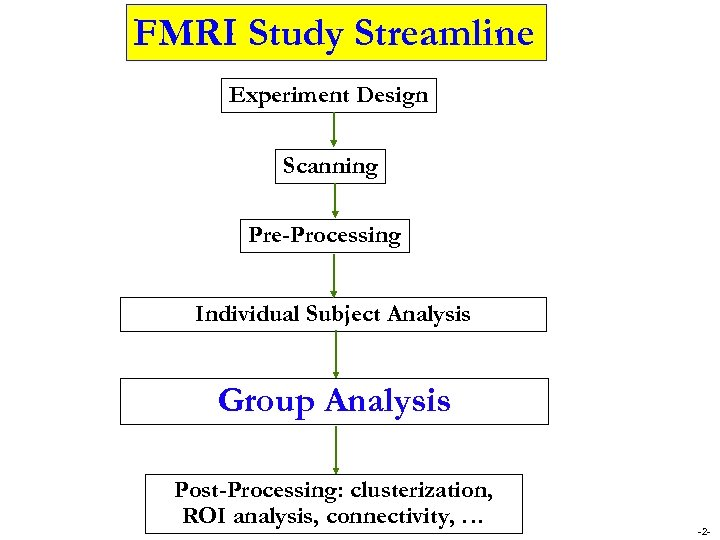FMRI Study Streamline Experiment Design Scanning Pre-Processing Individual Subject Analysis Group Analysis Post-Processing: clusterization, ROI analysis, connectivity, … -2 -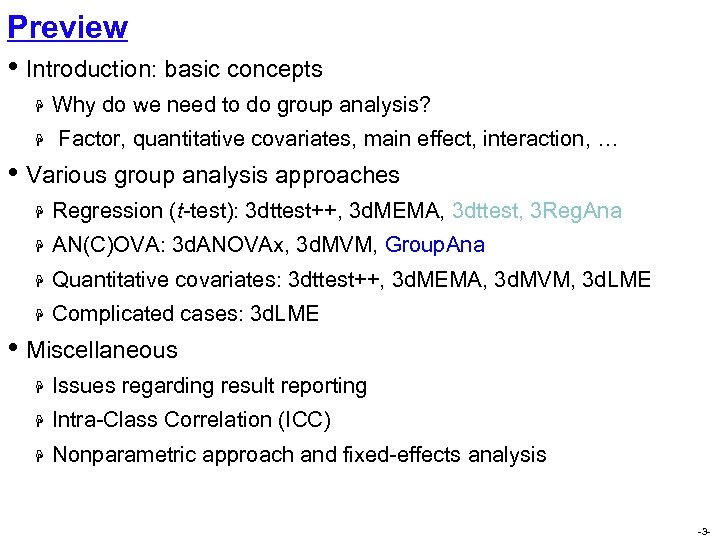Preview • Introduction: basic concepts H H Why do we need to do group analysis? Factor, quantitative covariates, main effect, interaction, … • Various group analysis approaches H Regression (t-test): 3 dttest++, 3 d. MEMA, 3 dttest, 3 Reg. Ana H AN(C)OVA: 3 d. ANOVAx, 3 d. MVM, Group. Ana H Quantitative covariates: 3 dttest++, 3 d. MEMA, 3 d. MVM, 3 d. LME H Complicated cases: 3 d. LME • Miscellaneous H Issues regarding result reporting H Intra-Class Correlation (ICC) H Nonparametric approach and fixed-effects analysis -3 -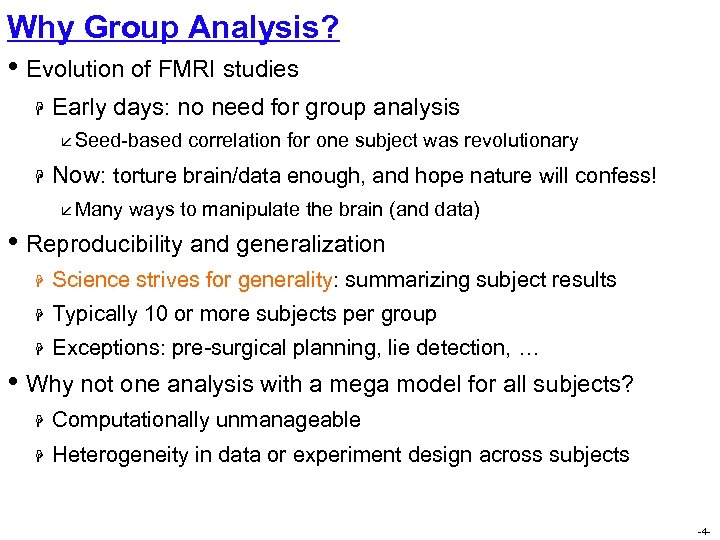Why Group Analysis? • Evolution of FMRI studies H Early days: no need for group analysis å Seed-based H correlation for one subject was revolutionary Now: torture brain/data enough, and hope nature will confess! å Many ways to manipulate the brain (and data) • Reproducibility and generalization H Science strives for generality: summarizing subject results H Typically 10 or more subjects per group H Exceptions: pre-surgical planning, lie detection, … • Why not one analysis with a mega model for all subjects? H Computationally unmanageable H Heterogeneity in data or experiment design across subjects -4 -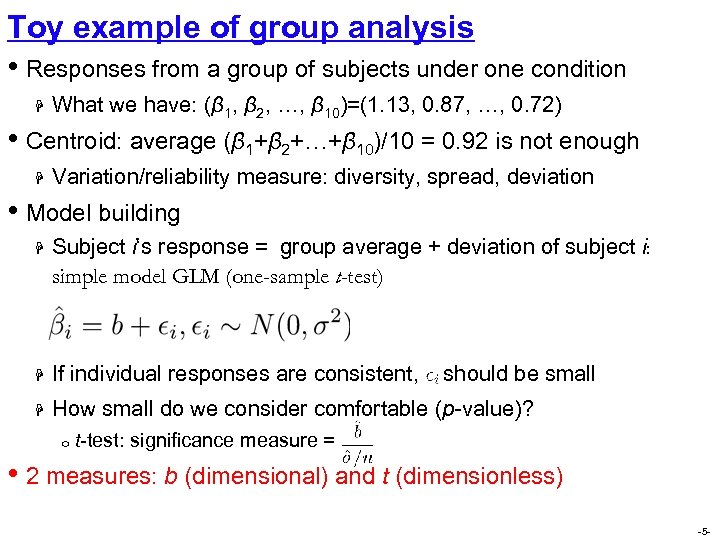Toy example of group analysis • Responses from a group of subjects under one condition H What we have: (β 1, β 2, …, β 10)=(1. 13, 0. 87, …, 0. 72) • Centroid: average (β 1+β 2+…+β 10)/10 = 0. 92 is not enough H Variation/reliability measure: diversity, spread, deviation • Model building H Subject i‘s response = group average + deviation of subject i: simple model GLM (one-sample t-test) H If individual responses are consistent, H How small do we consider comfortable (p-value)? o should be small t-test: significance measure = • 2 measures: b (dimensional) and t (dimensionless) -5 -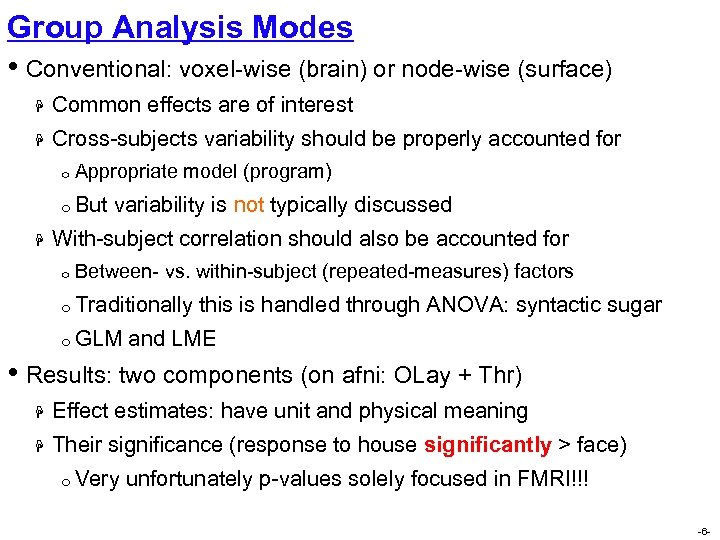Group Analysis Modes • Conventional: voxel-wise (brain) or node-wise (surface) H Common effects are of interest H Cross-subjects variability should be properly accounted for o Appropriate model (program) o But H variability is not typically discussed With-subject correlation should also be accounted for o Between- vs. within-subject (repeated-measures) factors o Traditionally o GLM this is handled through ANOVA: syntactic sugar and LME • Results: two components (on afni: OLay + Thr) H Effect estimates: have unit and physical meaning H Their significance (response to house significantly > face) o Very unfortunately p-values solely focused in FMRI!!! -6 -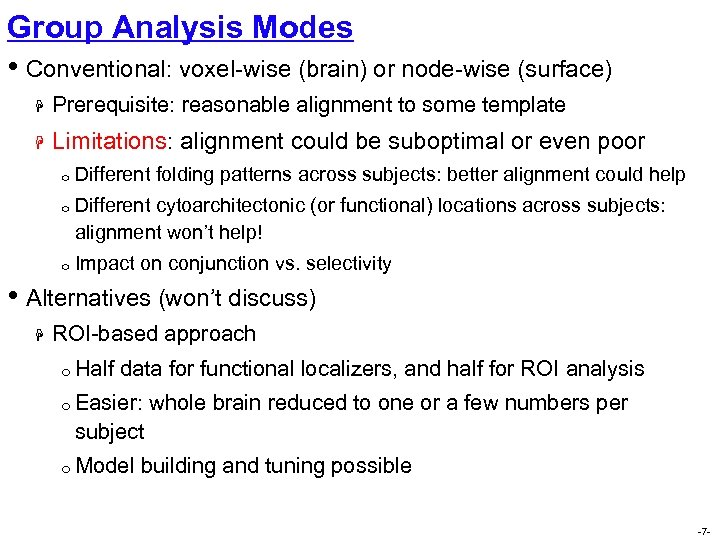Group Analysis Modes • Conventional: voxel-wise (brain) or node-wise (surface) H Prerequisite: reasonable alignment to some template H Limitations: alignment could be suboptimal or even poor o Different folding patterns across subjects: better alignment could help o Different cytoarchitectonic (or functional) locations across subjects: alignment won’t help! o Impact on conjunction vs. selectivity • Alternatives (won’t discuss) H ROI-based approach o Half data for functional localizers, and half for ROI analysis o Easier: whole brain reduced to one or a few numbers per subject o Model building and tuning possible -7 -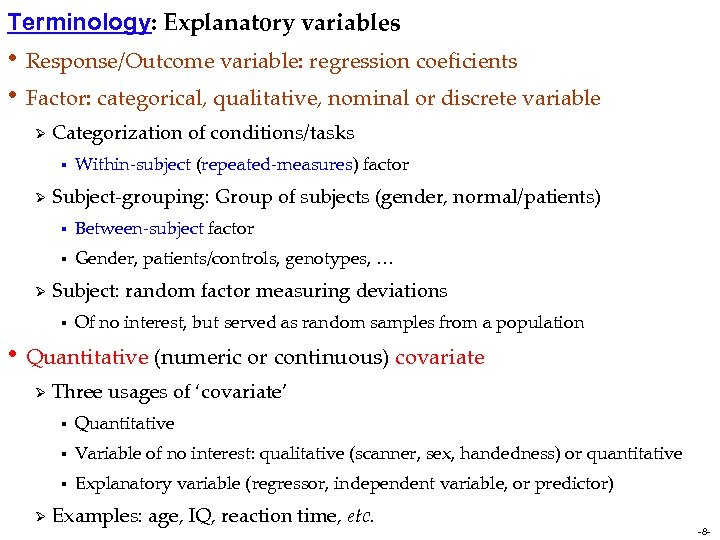Terminology: Explanatory variables • Response/Outcome variable: regression coeficients • Factor: categorical, qualitative, nominal or discrete variable Ø Categorization of conditions/tasks § Ø Within-subject (repeated-measures) factor Subject-grouping: Group of subjects (gender, normal/patients) § § Ø Between-subject factor Gender, patients/controls, genotypes, … Subject: random factor measuring deviations § Of no interest, but served as random samples from a population • Quantitative (numeric or continuous) covariate Ø Three usages of ‘covariate’ § § Variable of no interest: qualitative (scanner, sex, handedness) or quantitative § Ø Quantitative Explanatory variable (regressor, independent variable, or predictor) Examples: age, IQ, reaction time, etc. -8 -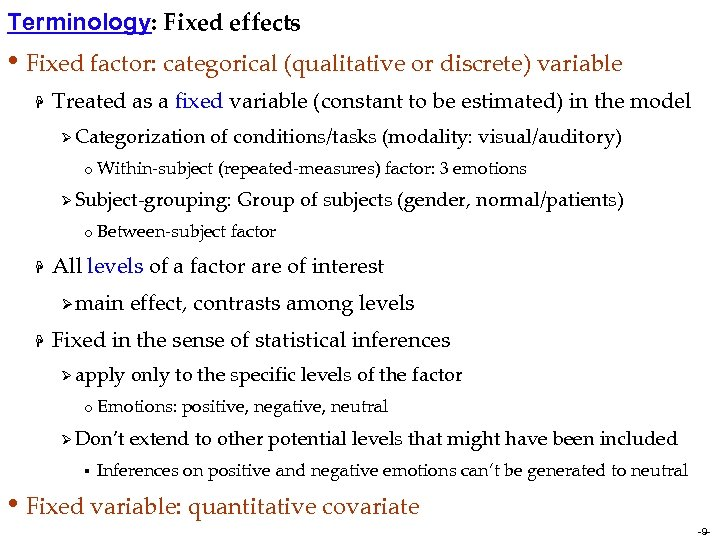Terminology: Fixed effects • Fixed factor: categorical (qualitative or discrete) variable H Treated as a fixed variable (constant to be estimated) in the model Ø Categorization of conditions/tasks (modality: visual/auditory) o Within-subject (repeated-measures) factor: 3 emotions Ø Subject-grouping: o Between-subject H factor All levels of a factor are of interest Ø main H Group of subjects (gender, normal/patients) effect, contrasts among levels Fixed in the sense of statistical inferences Ø apply only to the specific levels of the factor o Emotions: Ø Don’t § positive, negative, neutral extend to other potential levels that might have been included Inferences on positive and negative emotions can’t be generated to neutral • Fixed variable: quantitative covariate -9 -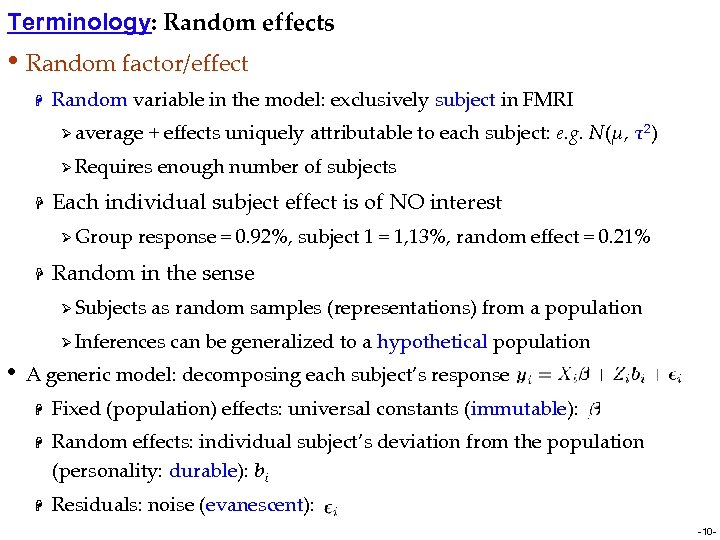Terminology: Random effects • Random factor/effect H Random variable in the model: exclusively subject in FMRI Ø average + effects uniquely attributable to each subject: e. g. N(μ, τ2) Ø Requires H Each individual subject effect is of NO interest Ø Group H enough number of subjects response = 0. 92%, subject 1 = 1, 13%, random effect = 0. 21% Random in the sense Ø Subjects as random samples (representations) from a population Ø Inferences • can be generalized to a hypothetical population A generic model: decomposing each subject’s response H H H Fixed (population) effects: universal constants (immutable): Random effects: individual subject’s deviation from the population (personality: durable): bi Residuals: noise (evanescent): -10 -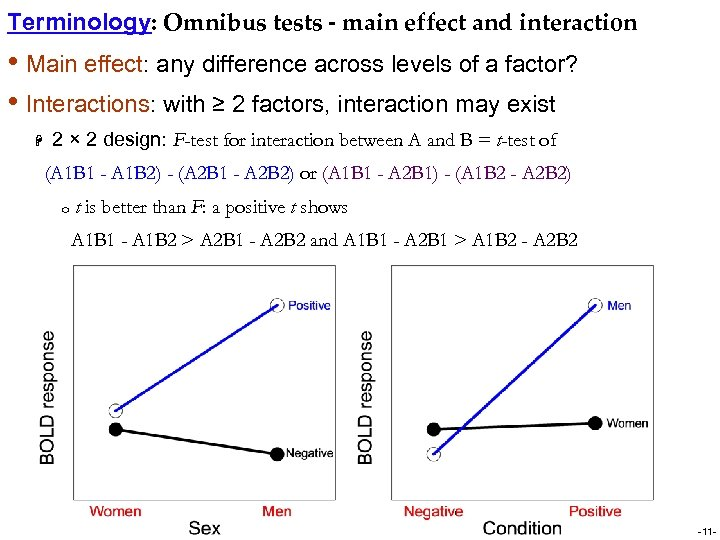Terminology: Omnibus tests - main effect and interaction • Main effect: any difference across levels of a factor? • Interactions: with ≥ 2 factors, interaction may exist H 2 × 2 design: F-test for interaction between A and B = t-test of (A 1 B 1 - A 1 B 2) - (A 2 B 1 - A 2 B 2) or (A 1 B 1 - A 2 B 1) - (A 1 B 2 - A 2 B 2) o t is better than F: a positive t shows A 1 B 1 - A 1 B 2 > A 2 B 1 - A 2 B 2 and A 1 B 1 - A 2 B 1 > A 1 B 2 - A 2 B 2 -11 -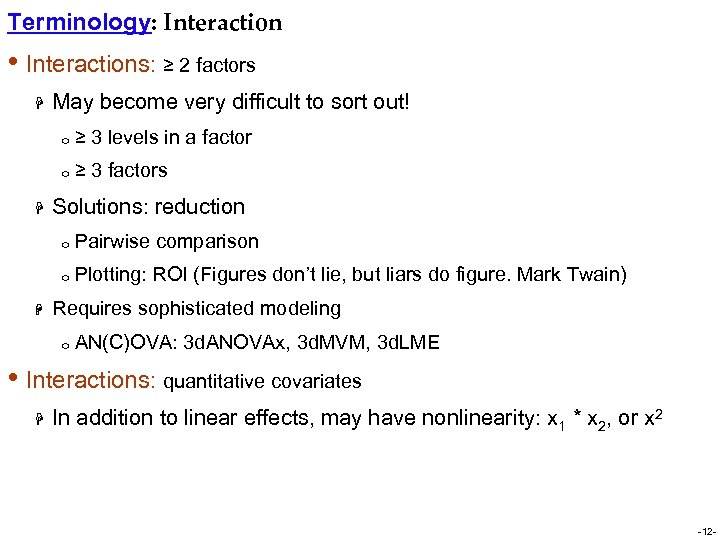Terminology: Interaction • Interactions: ≥ 2 factors H May become very difficult to sort out! o o H ≥ 3 levels in a factor ≥ 3 factors Solutions: reduction o o H Pairwise comparison Plotting: ROI (Figures don’t lie, but liars do figure. Mark Twain) Requires sophisticated modeling o AN(C)OVA: 3 d. ANOVAx, 3 d. MVM, 3 d. LME • Interactions: quantitative covariates H In addition to linear effects, may have nonlinearity: x 1 * x 2, or x 2 -12 -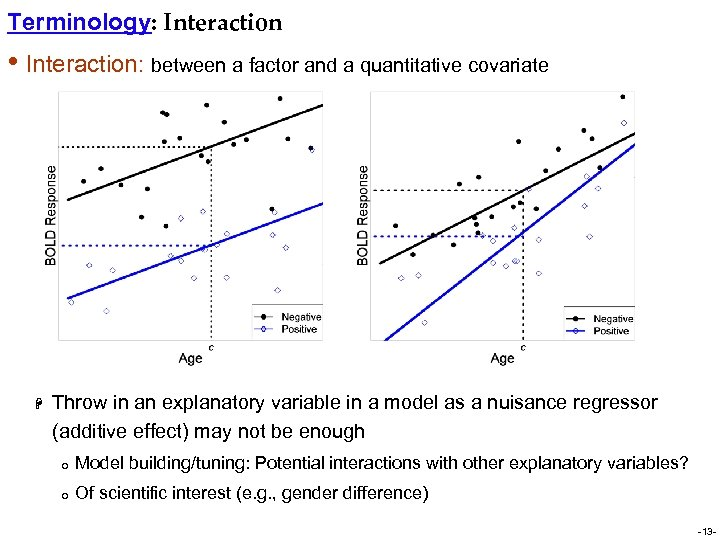Terminology: Interaction • Interaction: between a factor and a quantitative covariate H Throw in an explanatory variable in a model as a nuisance regressor (additive effect) may not be enough o Model building/tuning: Potential interactions with other explanatory variables? o Of scientific interest (e. g. , gender difference) -13 -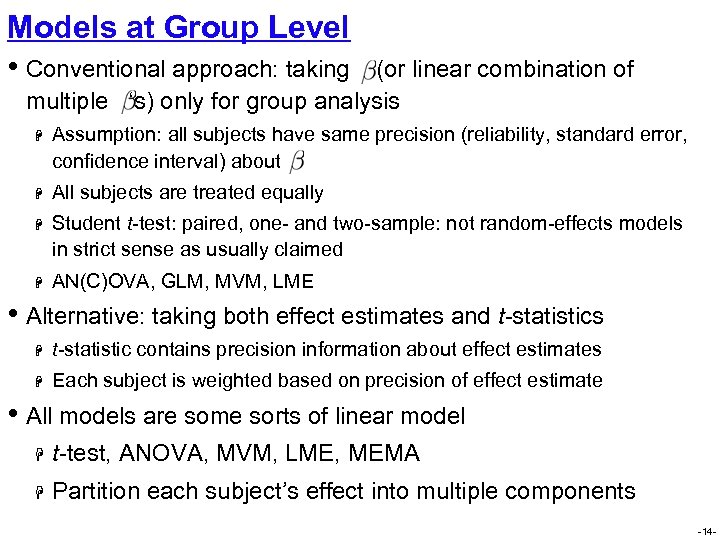Models at Group Level • Conventional approach: taking (or linear combination of multiple ‘s) only for group analysis H Assumption: all subjects have same precision (reliability, standard error, confidence interval) about H All subjects are treated equally H Student t-test: paired, one- and two-sample: not random-effects models in strict sense as usually claimed H AN(C)OVA, GLM, MVM, LME • Alternative: taking both effect estimates and t-statistics H t-statistic contains precision information about effect estimates H Each subject is weighted based on precision of effect estimate • All models are some sorts of linear model H t-test, ANOVA, MVM, LME, MEMA H Partition each subject’s effect into multiple components -14 -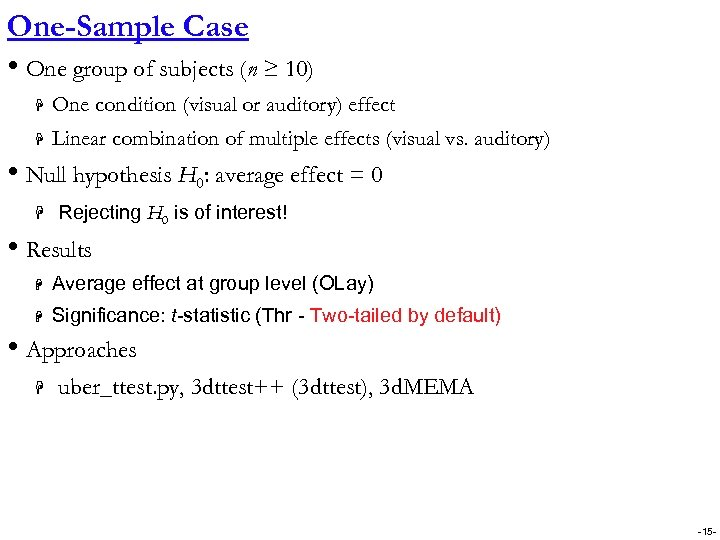One-Sample Case • One group of subjects (n ≥ 10) H One condition (visual or auditory) effect H Linear combination of multiple effects (visual vs. auditory) • Null hypothesis H 0: average effect = 0 H Rejecting H 0 is of interest! • Results H Average effect at group level (OLay) H Significance: t-statistic (Thr - Two-tailed by default) • Approaches H uber_ttest. py, 3 dttest++ (3 dttest), 3 d. MEMA -15 -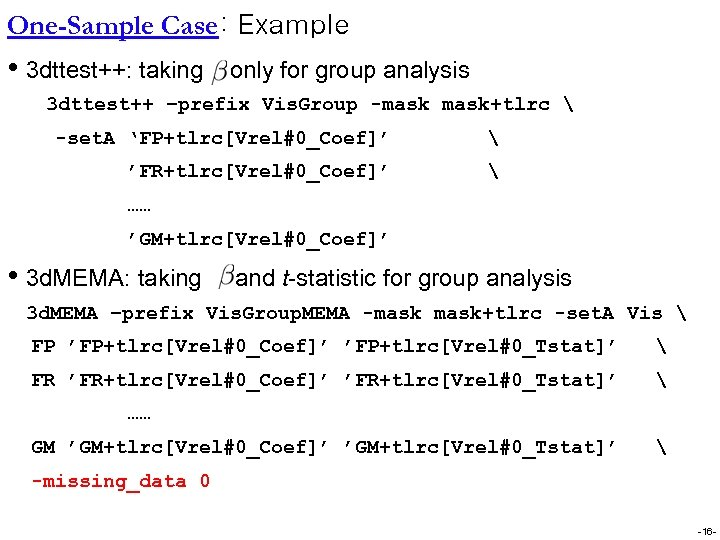One-Sample Case: Example • 3 dttest++: taking only for group analysis 3 dttest++ –prefix Vis. Group -mask+tlrc -set. A ‘FP+tlrc[Vrel#0_Coef]’ ’FR+tlrc[Vrel#0_Coef]’ …… ’GM+tlrc[Vrel#0_Coef]’ • 3 d. MEMA: taking and t-statistic for group analysis 3 d. MEMA –prefix Vis. Group. MEMA -mask+tlrc -set. A Vis FP ’FP+tlrc[Vrel#0_Coef]’ ’FP+tlrc[Vrel#0_Tstat]’ FR ’FR+tlrc[Vrel#0_Coef]’ ’FR+tlrc[Vrel#0_Tstat]’ …… GM ’GM+tlrc[Vrel#0_Coef]’ ’GM+tlrc[Vrel#0_Tstat]’ -missing_data 0 -16 -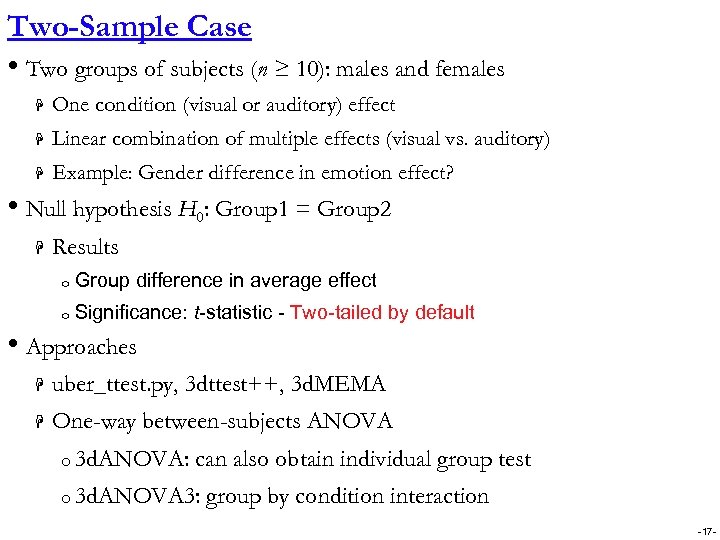Two-Sample Case • Two groups of subjects (n ≥ 10): males and females H One condition (visual or auditory) effect H Linear combination of multiple effects (visual vs. auditory) H Example: Gender difference in emotion effect? • Null hypothesis H 0: Group 1 = Group 2 H Results o Group difference in average effect o Significance: t-statistic - Two-tailed by default • Approaches H uber_ttest. py, 3 dttest++, 3 d. MEMA H One-way between-subjects ANOVA o 3 d. ANOVA: can also obtain individual group test o 3 d. ANOVA 3: group by condition interaction -17 -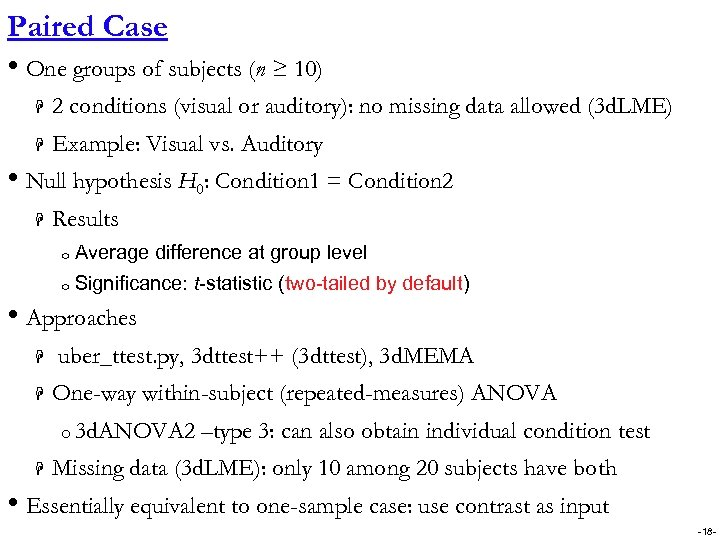Paired Case • One groups of subjects (n ≥ 10) H 2 conditions (visual or auditory): no missing data allowed (3 d. LME) H Example: Visual vs. Auditory • Null hypothesis H 0: Condition 1 = Condition 2 H Results o Average difference at group level o Significance: t-statistic (two-tailed by default) • Approaches H H uber_ttest. py, 3 dttest++ (3 dttest), 3 d. MEMA One-way within-subject (repeated-measures) ANOVA o 3 d. ANOVA 2 H –type 3: can also obtain individual condition test Missing data (3 d. LME): only 10 among 20 subjects have both • Essentially equivalent to one-sample case: use contrast as input -18 -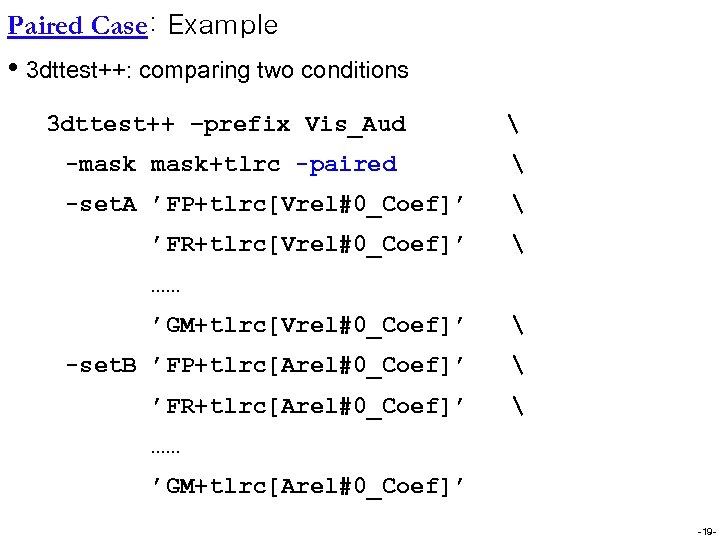Paired Case: Example • 3 dttest++: comparing two conditions 3 dttest++ –prefix Vis_Aud -mask+tlrc -paired -set. A ’FP+tlrc[Vrel#0_Coef]’ ’FR+tlrc[Vrel#0_Coef]’ …… ’GM+tlrc[Vrel#0_Coef]’ -set. B ’FP+tlrc[Arel#0_Coef]’ ’FR+tlrc[Arel#0_Coef]’ …… ’GM+tlrc[Arel#0_Coef]’ -19 -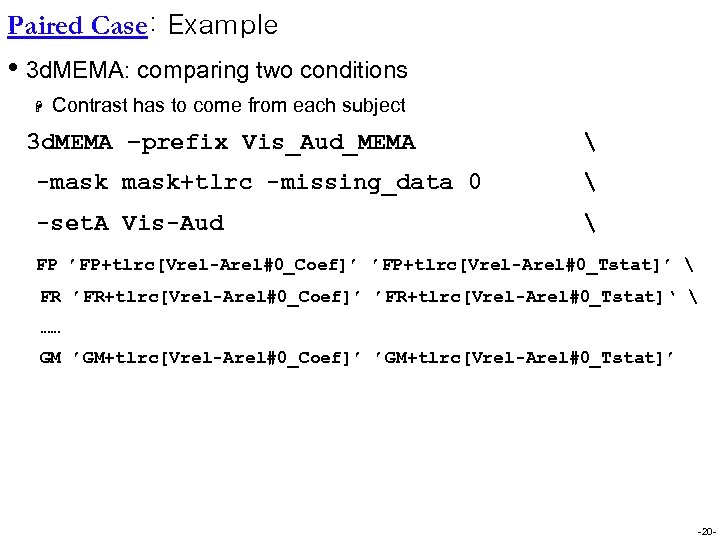Paired Case: Example • 3 d. MEMA: comparing two conditions H Contrast has to come from each subject 3 d. MEMA –prefix Vis_Aud_MEMA -mask+tlrc -missing_data 0 -set. A Vis-Aud FP ’FP+tlrc[Vrel-Arel#0_Coef]’ ’FP+tlrc[Vrel-Arel#0_Tstat]’ FR ’FR+tlrc[Vrel-Arel#0_Coef]’ ’FR+tlrc[Vrel-Arel#0_Tstat]‘ …… GM ’GM+tlrc[Vrel-Arel#0_Coef]’ ’GM+tlrc[Vrel-Arel#0_Tstat]’ -20 -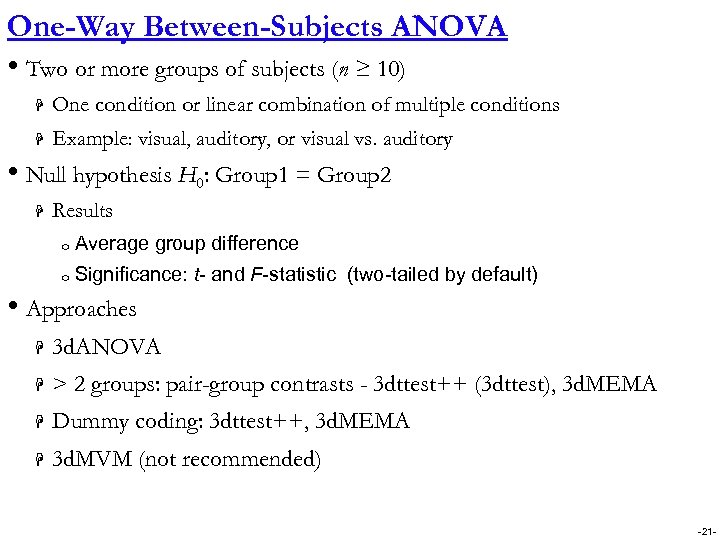One-Way Between-Subjects ANOVA • Two or more groups of subjects (n ≥ 10) H One condition or linear combination of multiple conditions H Example: visual, auditory, or visual vs. auditory • Null hypothesis H 0: Group 1 = Group 2 H Results o Average group difference o Significance: t- and F-statistic (two-tailed by default) • Approaches H 3 d. ANOVA H > 2 groups: pair-group contrasts - 3 dttest++ (3 dttest), 3 d. MEMA H Dummy coding: 3 dttest++, 3 d. MEMA H 3 d. MVM (not recommended) -21 -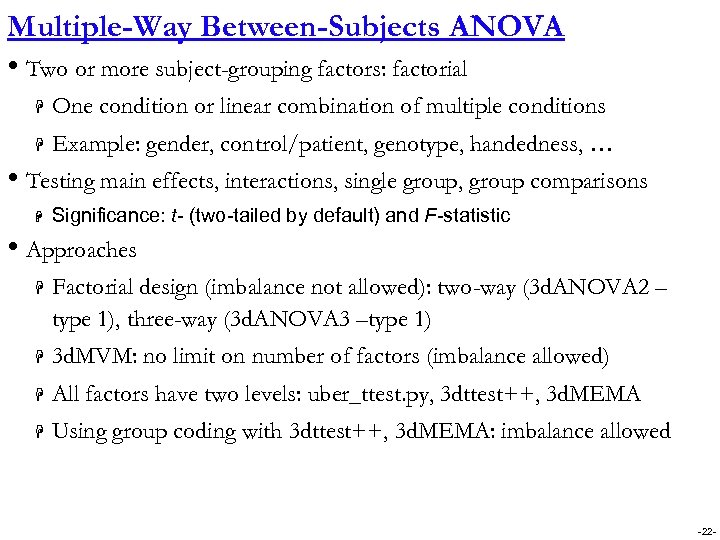Multiple-Way Between-Subjects ANOVA • Two or more subject-grouping factors: factorial H One condition or linear combination of multiple conditions H Example: gender, control/patient, genotype, handedness, … • Testing main effects, interactions, single group, group comparisons H Significance: t- (two-tailed by default) and F-statistic • Approaches H Factorial design (imbalance not allowed): two-way (3 d. ANOVA 2 – type 1), three-way (3 d. ANOVA 3 –type 1) H 3 d. MVM: no limit on number of factors (imbalance allowed) H All factors have two levels: uber_ttest. py, 3 dttest++, 3 d. MEMA H Using group coding with 3 dttest++, 3 d. MEMA: imbalance allowed -22 -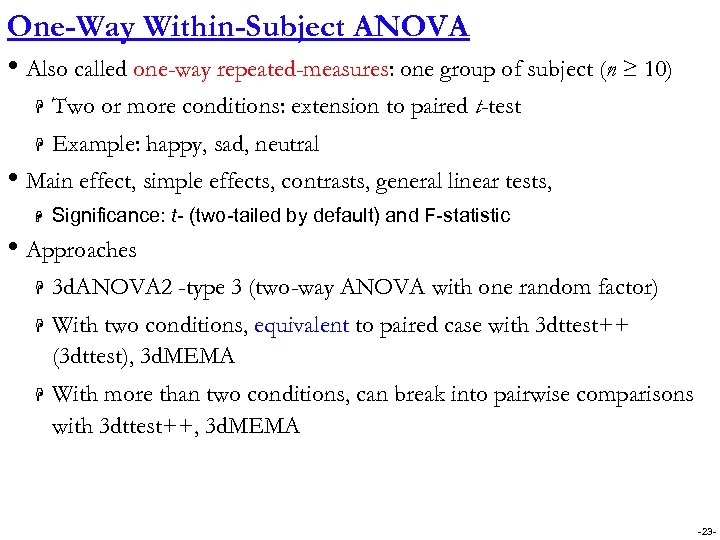One-Way Within-Subject ANOVA • Also called one-way repeated-measures: one group of subject (n ≥ 10) H Two or more conditions: extension to paired t-test H Example: happy, sad, neutral • Main effect, simple effects, contrasts, general linear tests, H Significance: t- (two-tailed by default) and F-statistic • Approaches H 3 d. ANOVA 2 -type 3 (two-way ANOVA with one random factor) H With two conditions, equivalent to paired case with 3 dttest++ (3 dttest), 3 d. MEMA H With more than two conditions, can break into pairwise comparisons with 3 dttest++, 3 d. MEMA -23 -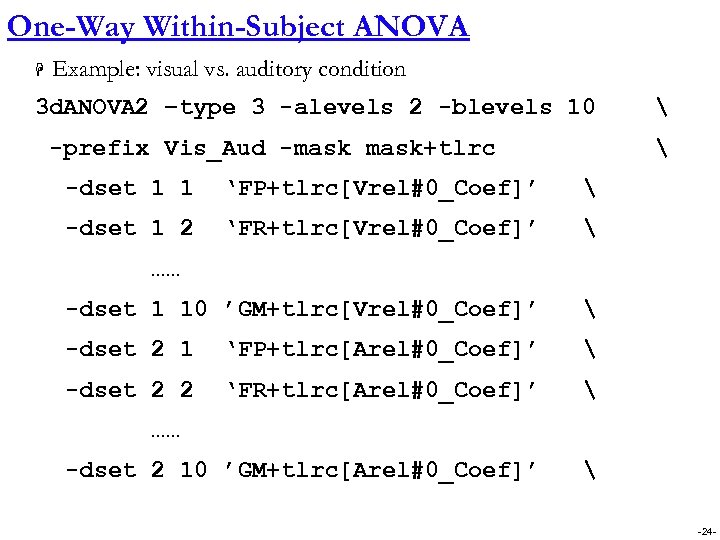One-Way Within-Subject ANOVA H Example: visual vs. auditory condition 3 d. ANOVA 2 –type 3 -alevels 2 -blevels 10 -prefix Vis_Aud -mask+tlrc -dset 1 1 ‘FP+tlrc[Vrel#0_Coef]’ -dset 1 2 ‘FR+tlrc[Vrel#0_Coef]’ -dset 1 10 ’GM+tlrc[Vrel#0_Coef]’ -dset 2 1 ‘FP+tlrc[Arel#0_Coef]’ -dset 2 2 ‘FR+tlrc[Arel#0_Coef]’ -dset 2 10 ’GM+tlrc[Arel#0_Coef]’ …… …… -24 -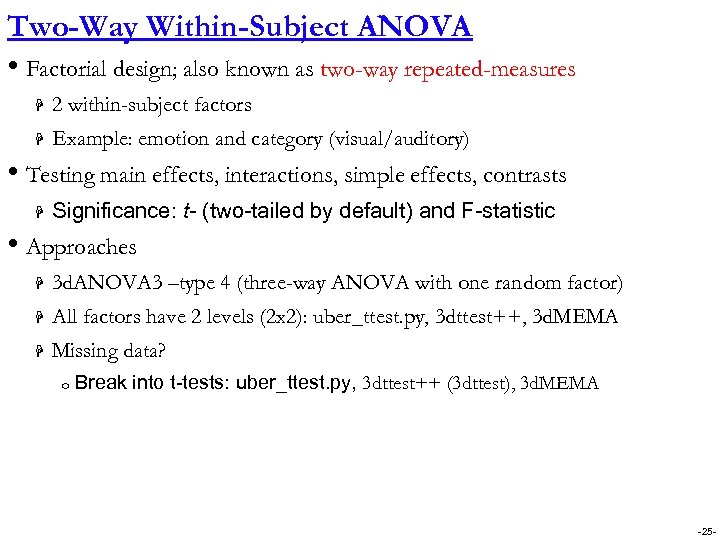Two-Way Within-Subject ANOVA • Factorial design; also known as two-way repeated-measures H 2 within-subject factors H Example: emotion and category (visual/auditory) • Testing main effects, interactions, simple effects, contrasts H Significance: t- (two-tailed by default) and F-statistic • Approaches H 3 d. ANOVA 3 –type 4 (three-way ANOVA with one random factor) H All factors have 2 levels (2 x 2): uber_ttest. py, 3 dttest++, 3 d. MEMA H Missing data? o Break into t-tests: uber_ttest. py, 3 dttest++ (3 dttest), 3 d. MEMA -25 -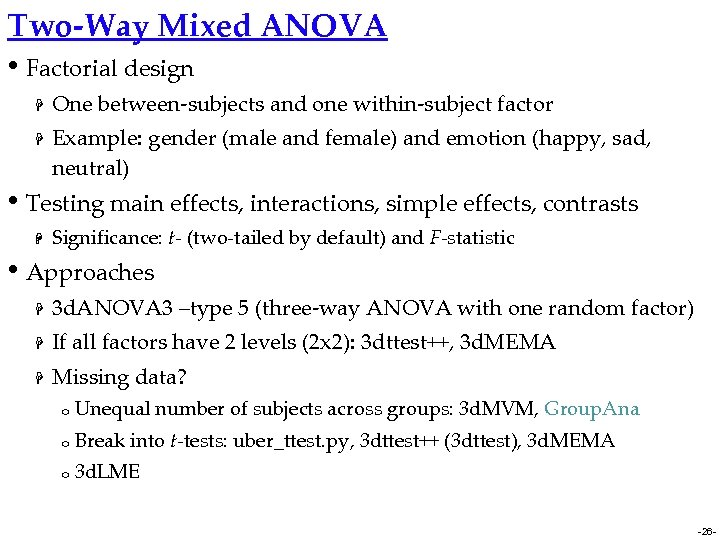Two-Way Mixed ANOVA • Factorial design H H One between-subjects and one within-subject factor Example: gender (male and female) and emotion (happy, sad, neutral) • Testing main effects, interactions, simple effects, contrasts H Significance: t- (two-tailed by default) and F-statistic • Approaches H 3 d. ANOVA 3 –type 5 (three-way ANOVA with one random factor) H If all factors have 2 levels (2 x 2): 3 dttest++, 3 d. MEMA H Missing data? o Unequal number of subjects across groups: 3 d. MVM, Group. Ana o Break into t-tests: uber_ttest. py, 3 dttest++ (3 dttest), 3 d. MEMA o 3 d. LME -26 -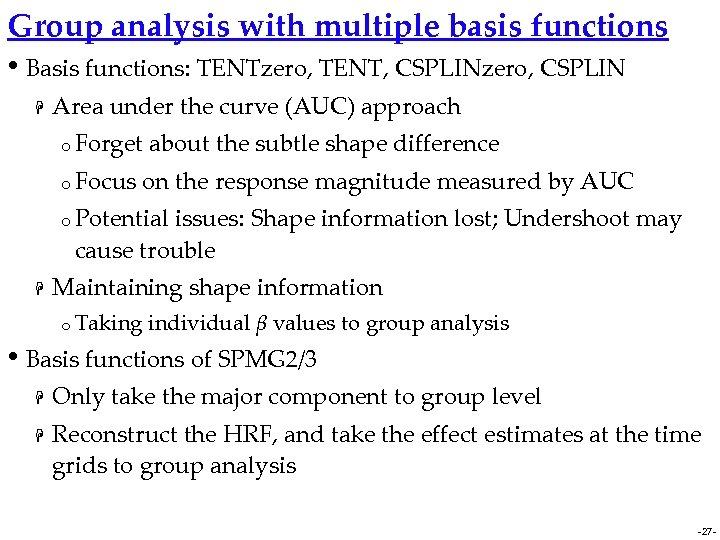Group analysis with multiple basis functions • Basis functions: TENTzero, TENT, CSPLINzero, CSPLIN H Area under the curve (AUC) approach o Forget o Focus about the subtle shape difference on the response magnitude measured by AUC o Potential issues: Shape information lost; Undershoot may cause trouble H Maintaining shape information o Taking individual β values to group analysis • Basis functions of SPMG 2/3 H H Only take the major component to group level Reconstruct the HRF, and take the effect estimates at the time grids to group analysis -27 -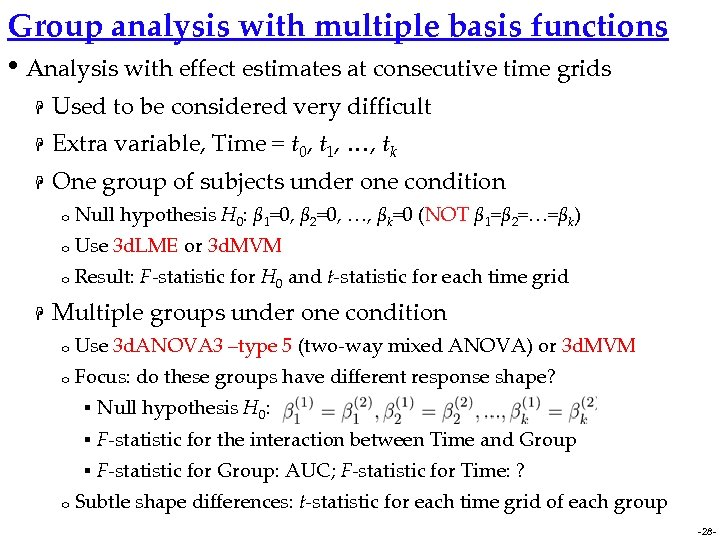Group analysis with multiple basis functions • Analysis with effect estimates at consecutive time grids H Used to be considered very difficult H Extra variable, Time = t 0, t 1, …, tk H One group of subjects under one condition o o Use 3 d. LME or 3 d. MVM o H Null hypothesis H 0: β 1=0, β 2=0, …, βk=0 (NOT β 1=β 2=…=βk) Result: F-statistic for H 0 and t-statistic for each time grid Multiple groups under one condition o Use 3 d. ANOVA 3 –type 5 (two-way mixed ANOVA) or 3 d. MVM o Focus: do these groups have different response shape? § § F-statistic for the interaction between Time and Group § o Null hypothesis H 0: F-statistic for Group: AUC; F-statistic for Time: ? Subtle shape differences: t-statistic for each time grid of each group -28 -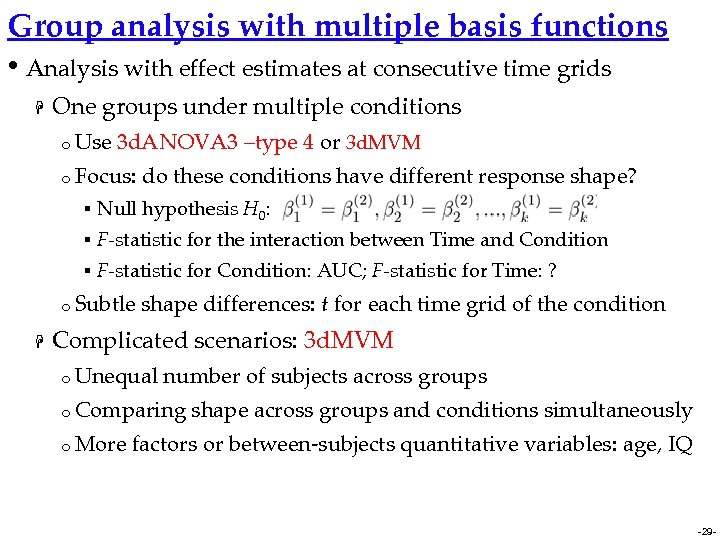Group analysis with multiple basis functions • Analysis with effect estimates at consecutive time grids H One groups under multiple conditions o Use 3 d. ANOVA 3 –type 4 or 3 d. MVM o Focus: do these conditions have different response shape? § Null hypothesis H 0: § F-statistic for the interaction between Time and Condition § F-statistic for Condition: AUC; F-statistic for Time: ? o Subtle H shape differences: t for each time grid of the condition Complicated scenarios: 3 d. MVM o Unequal number of subjects across groups o Comparing o More shape across groups and conditions simultaneously factors or between-subjects quantitative variables: age, IQ -29 -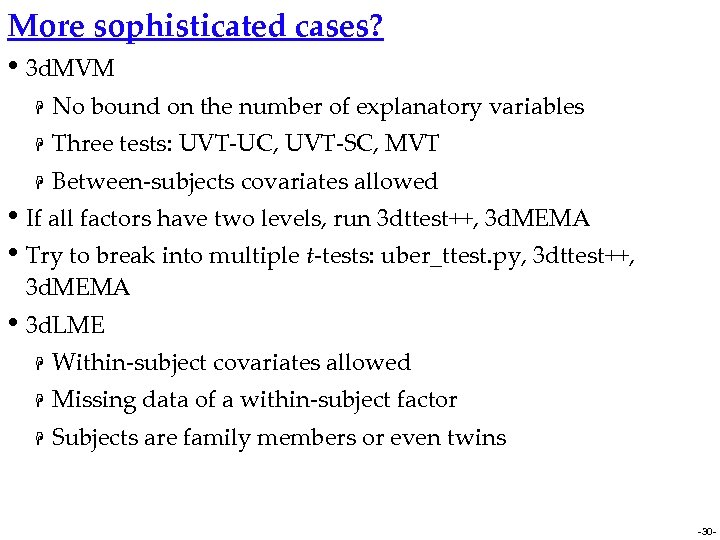More sophisticated cases? • 3 d. MVM H No bound on the number of explanatory variables H Three tests: UVT-UC, UVT-SC, MVT H Between-subjects covariates allowed • If all factors have two levels, run 3 dttest++, 3 d. MEMA • Try to break into multiple t-tests: uber_ttest. py, 3 dttest++, 3 d. MEMA • 3 d. LME H Within-subject covariates allowed H Missing data of a within-subject factor H Subjects are family members or even twins -30 -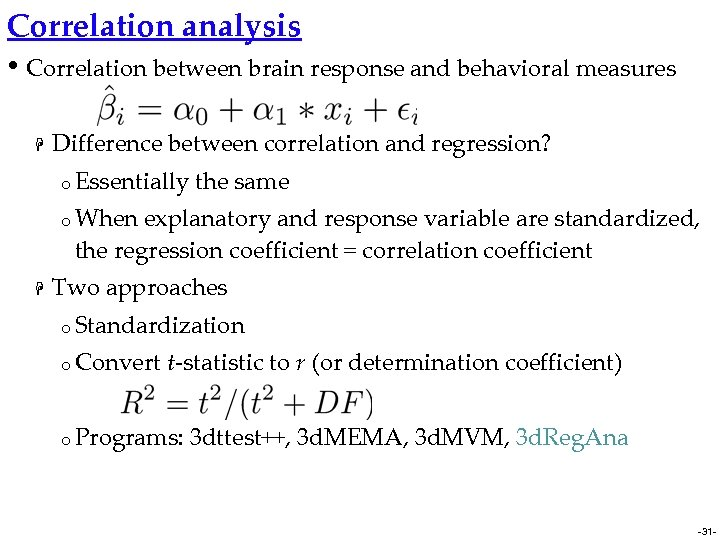Correlation analysis • Correlation between brain response and behavioral measures H Difference between correlation and regression? o Essentially the same o When explanatory and response variable are standardized, the regression coefficient = correlation coefficient H Two approaches o Standardization o Convert t-statistic to r (or determination coefficient) o Programs: 3 dttest++, 3 d. MEMA, 3 d. MVM, 3 d. Reg. Ana -31 -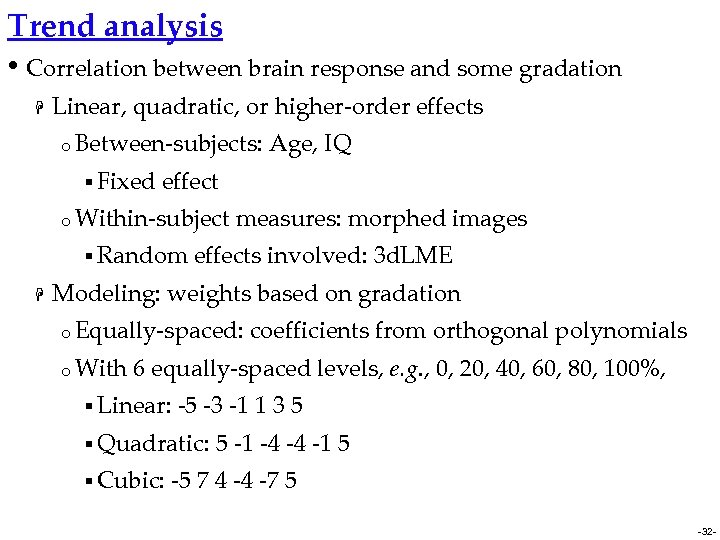Trend analysis • Correlation between brain response and some gradation H Linear, quadratic, or higher-order effects o Between-subjects: § Fixed effect o Within-subject § Random H Age, IQ measures: morphed images effects involved: 3 d. LME Modeling: weights based on gradation o Equally-spaced: o With coefficients from orthogonal polynomials 6 equally-spaced levels, e. g. , 0, 20, 40, 60, 80, 100%, § Linear: -5 -3 -1 1 3 5 § Quadratic: § Cubic: 5 -1 -4 -4 -1 5 -5 7 4 -4 -7 5 -32 -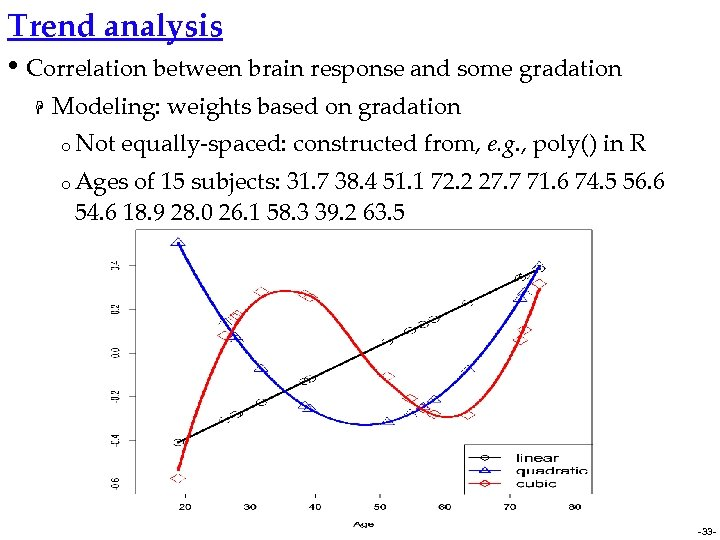Trend analysis • Correlation between brain response and some gradation H Modeling: weights based on gradation o Not equally-spaced: constructed from, e. g. , poly() in R o Ages of 15 subjects: 31. 7 38. 4 51. 1 72. 2 27. 7 71. 6 74. 5 56. 6 54. 6 18. 9 28. 0 26. 1 58. 3 39. 2 63. 5 -33 -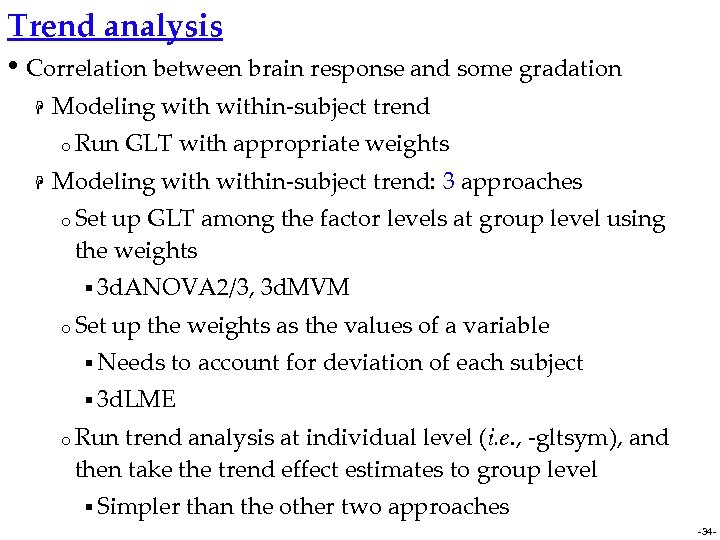Trend analysis • Correlation between brain response and some gradation H Modeling within-subject trend o Run H GLT with appropriate weights Modeling within-subject trend: 3 approaches o Set up GLT among the factor levels at group level using the weights § 3 d. ANOVA 2/3, o Set 3 d. MVM up the weights as the values of a variable § Needs to account for deviation of each subject § 3 d. LME o Run trend analysis at individual level (i. e. , -gltsym), and then take the trend effect estimates to group level § Simpler than the other two approaches -34 -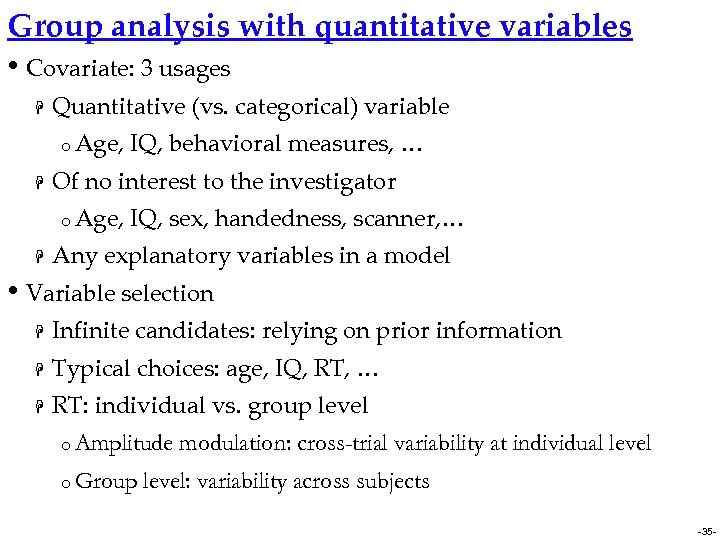Group analysis with quantitative variables • Covariate: 3 usages H Quantitative (vs. categorical) variable o Age, H Of no interest to the investigator o Age, H IQ, behavioral measures, … IQ, sex, handedness, scanner, … Any explanatory variables in a model • Variable selection H Infinite candidates: relying on prior information H Typical choices: age, IQ, RT, … H RT: individual vs. group level o Amplitude o Group modulation: cross-trial variability at individual level: variability across subjects -35 -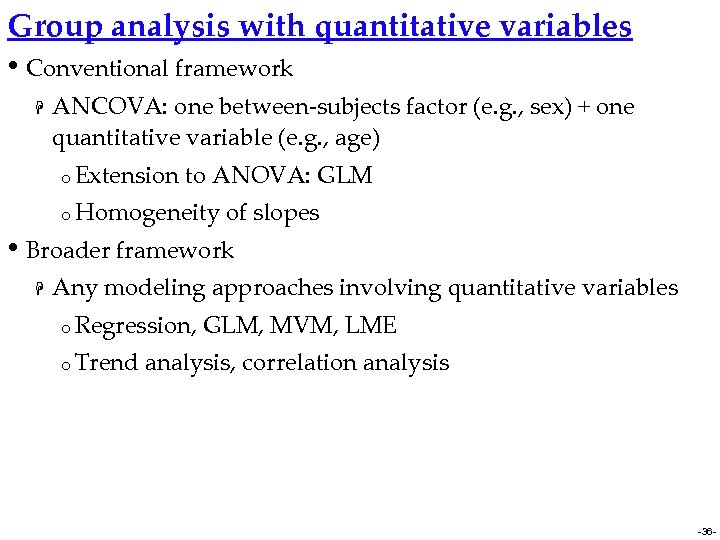Group analysis with quantitative variables • Conventional framework H ANCOVA: one between-subjects factor (e. g. , sex) + one quantitative variable (e. g. , age) o Extension to ANOVA: GLM o Homogeneity of slopes • Broader framework H Any modeling approaches involving quantitative variables o Regression, o Trend GLM, MVM, LME analysis, correlation analysis -36 -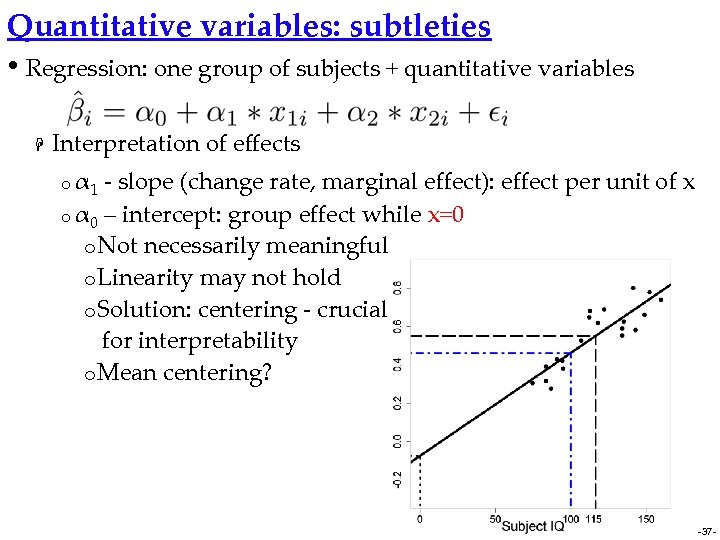Quantitative variables: subtleties • Regression: one group of subjects + quantitative variables H Interpretation of effects o α 1 - slope (change rate, marginal effect): effect per unit of x o α 0 – intercept: group effect while x=0 o Not necessarily meaningful o Linearity may not hold o Solution: centering - crucial for interpretability o Mean centering? -37 -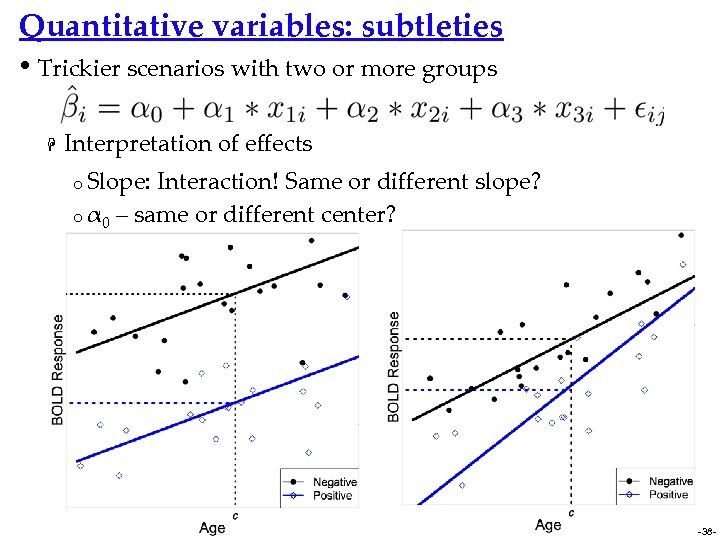Quantitative variables: subtleties • Trickier scenarios with two or more groups H Interpretation of effects o Slope: Interaction! Same or different slope? o α 0 – same or different center? -38 -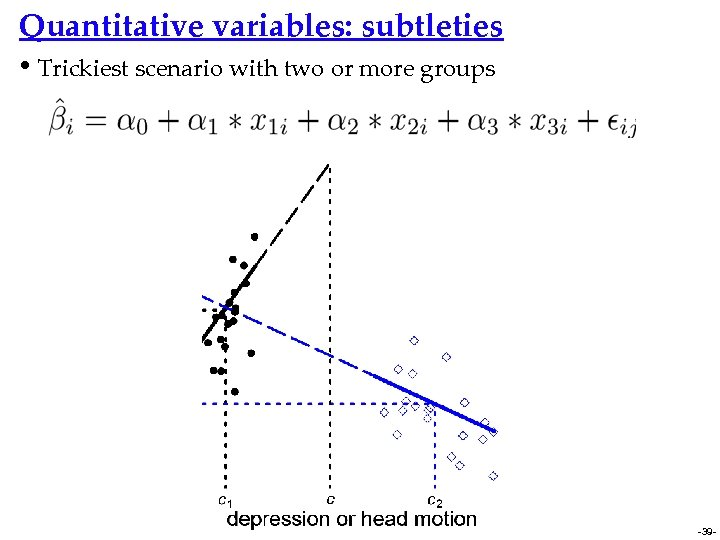Quantitative variables: subtleties • Trickiest scenario with two or more groups -39 -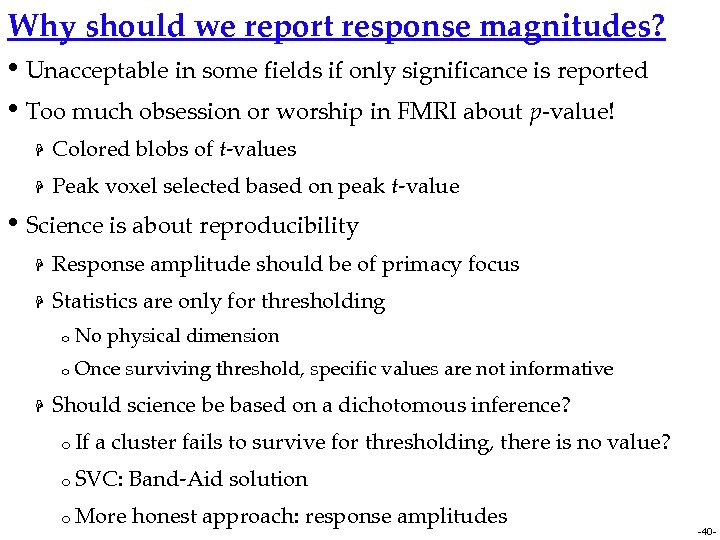Why should we report response magnitudes? • Unacceptable in some fields if only significance is reported • Too much obsession or worship in FMRI about p-value! H Colored blobs of t-values H Peak voxel selected based on peak t-value • Science is about reproducibility H Response amplitude should be of primacy focus H Statistics are only for thresholding o o H No physical dimension Once surviving threshold, specific values are not informative Should science be based on a dichotomous inference? o If a cluster fails to survive for thresholding, there is no value? o SVC: Band-Aid solution o More honest approach: response amplitudes -40 -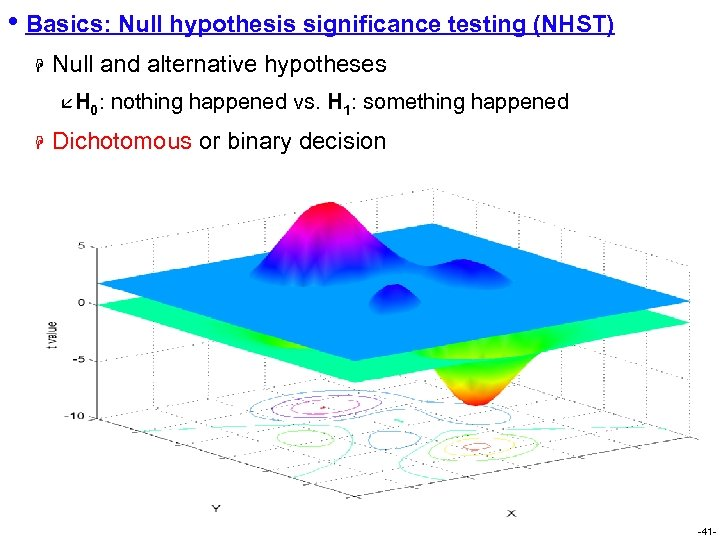• Basics: Null hypothesis significance testing (NHST) H Null and alternative hypotheses å H 0: H nothing happened vs. H 1: something happened Dichotomous or binary decision -41 -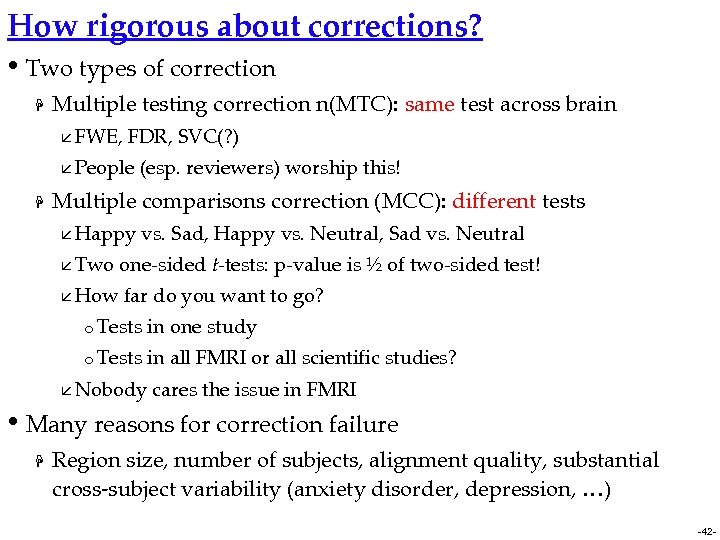How rigorous about corrections? • Two types of correction H Multiple testing correction n(MTC): same test across brain å FWE, FDR, SVC(? ) å People H (esp. reviewers) worship this! Multiple comparisons correction (MCC): different tests å Happy å Two å How vs. Sad, Happy vs. Neutral, Sad vs. Neutral one-sided t-tests: p-value is ½ of two-sided test! far do you want to go? o Tests in one study o Tests in all FMRI or all scientific studies? å Nobody cares the issue in FMRI • Many reasons for correction failure H Region size, number of subjects, alignment quality, substantial cross-subject variability (anxiety disorder, depression, …) -42 -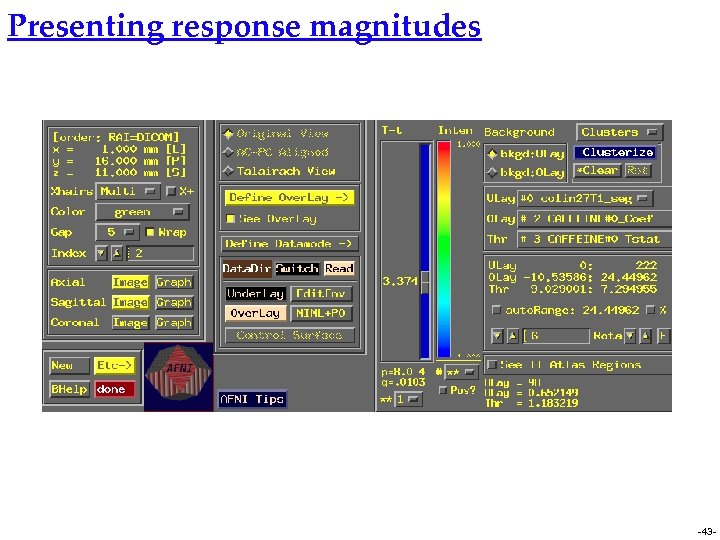Presenting response magnitudes -43 -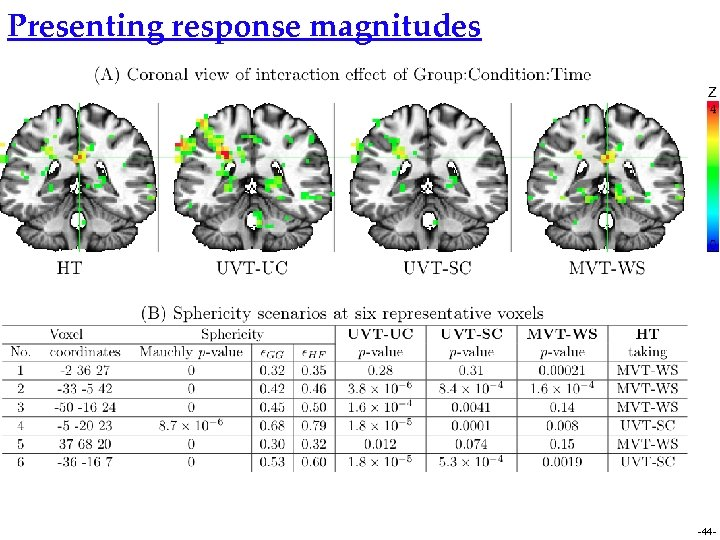Presenting response magnitudes -44 -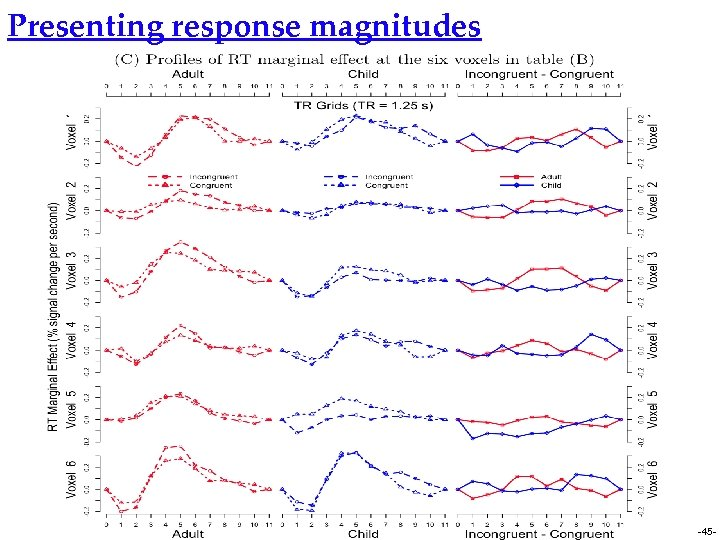Presenting response magnitudes -45 -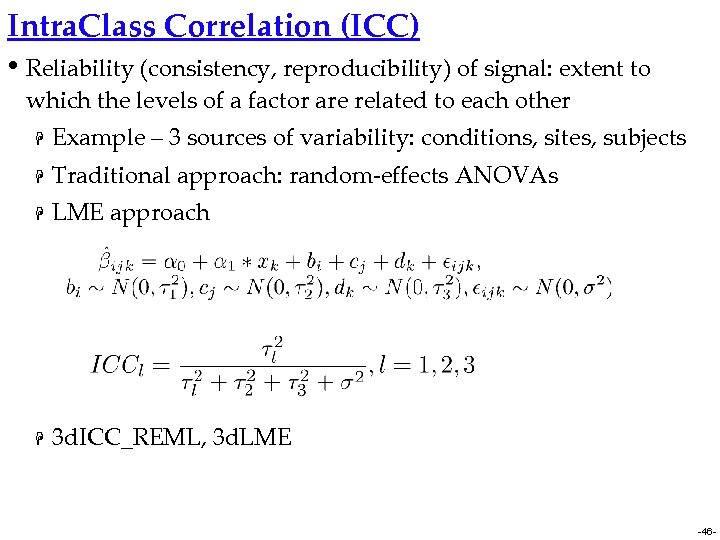Intra. Class Correlation (ICC) • Reliability (consistency, reproducibility) of signal: extent to which the levels of a factor are related to each other H Example – 3 sources of variability: conditions, sites, subjects H Traditional approach: random-effects ANOVAs H LME approach H 3 d. ICC_REML, 3 d. LME -46 -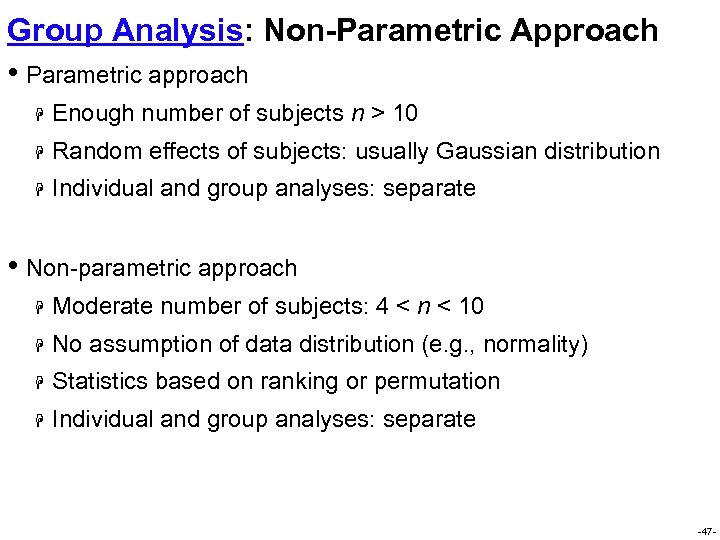Group Analysis: Non-Parametric Approach • Parametric approach H Enough number of subjects n > 10 H Random effects of subjects: usually Gaussian distribution H Individual and group analyses: separate • Non-parametric approach H Moderate number of subjects: 4 < n < 10 H No assumption of data distribution (e. g. , normality) H Statistics based on ranking or permutation H Individual and group analyses: separate -47 -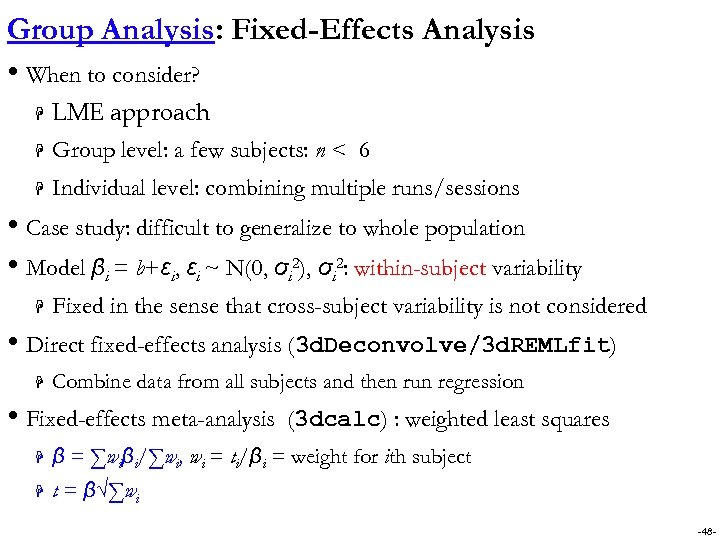Group Analysis: Fixed-Effects Analysis • When to consider? H LME approach H Group level: a few subjects: n < 6 H Individual level: combining multiple runs/sessions • Case study: difficult to generalize to whole population • Model βi = b+εi, εi ~ N(0, σi 2), σi 2: within-subject variability H Fixed in the sense that cross-subject variability is not considered • Direct fixed-effects analysis (3 d. Deconvolve/3 d. REMLfit) H Combine data from all subjects and then run regression • Fixed-effects meta-analysis (3 dcalc) : weighted least squares H β = ∑wiβi/∑wi, wi = ti/βi = weight for ith subject H t = β√∑wi -48 -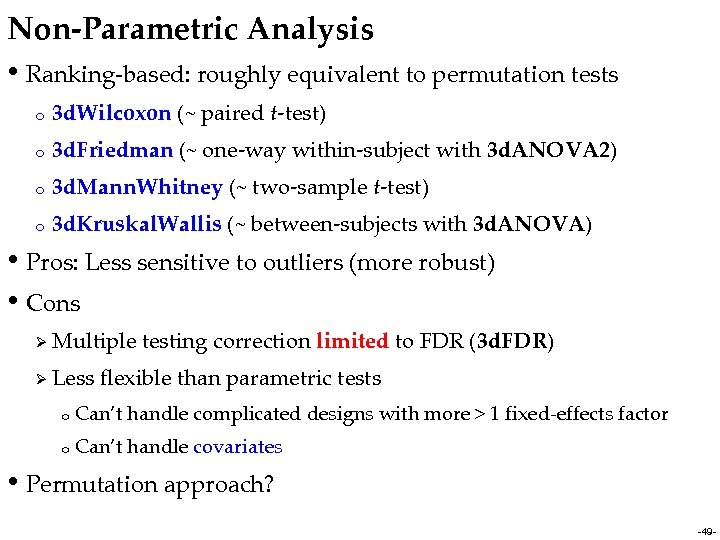Non-Parametric Analysis • Ranking-based: roughly equivalent to permutation tests o 3 d. Wilcoxon (~ paired t-test) o 3 d. Friedman (~ one-way within-subject with 3 d. ANOVA 2) o 3 d. Mann. Whitney (~ two-sample t-test) o 3 d. Kruskal. Wallis (~ between-subjects with 3 d. ANOVA) • Pros: Less sensitive to outliers (more robust) • Cons Ø Multiple testing correction limited to FDR (3 d. FDR) Ø Less flexible than parametric tests o Can’t handle complicated designs with more > 1 fixed-effects factor o Can’t handle covariates • Permutation approach? -49 -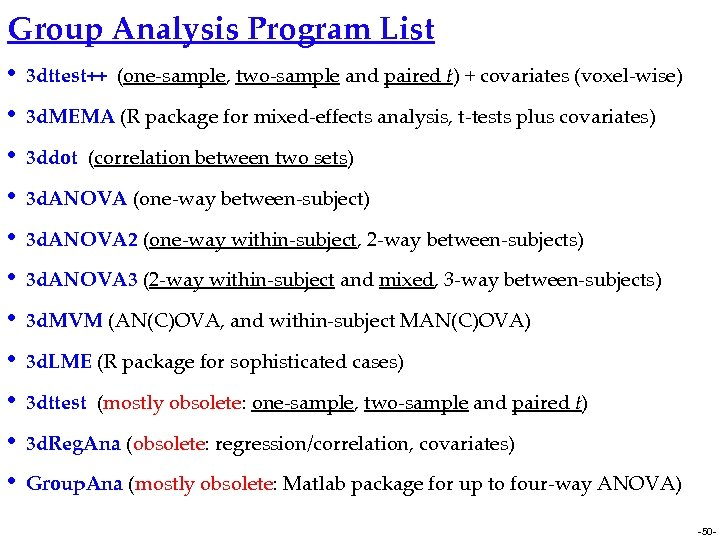Group Analysis Program List • 3 dttest++ (one-sample, two-sample and paired t) + covariates (voxel-wise) • 3 d. MEMA (R package for mixed-effects analysis, t-tests plus covariates) • 3 ddot (correlation between two sets) • 3 d. ANOVA (one-way between-subject) • 3 d. ANOVA 2 (one-way within-subject, 2 -way between-subjects) • 3 d. ANOVA 3 (2 -way within-subject and mixed, 3 -way between-subjects) • 3 d. MVM (AN(C)OVA, and within-subject MAN(C)OVA) • 3 d. LME (R package for sophisticated cases) • 3 dttest (mostly obsolete: one-sample, two-sample and paired t) • 3 d. Reg. Ana (obsolete: regression/correlation, covariates) • Group. Ana (mostly obsolete: Matlab package for up to four-way ANOVA) -50 -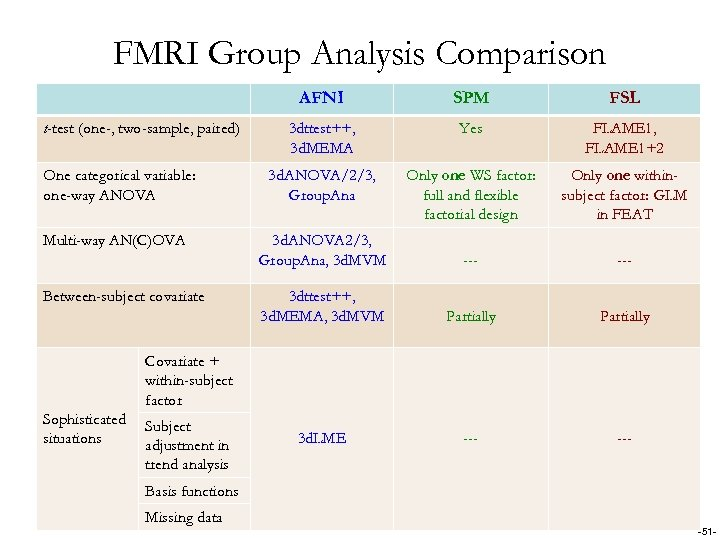FMRI Group Analysis Comparison AFNI t-test (one-, two-sample, paired) One categorical variable: one-way ANOVA Multi-way AN(C)OVA Between-subject covariate SPM FSL 3 dttest++, 3 d. MEMA Yes FLAME 1, FLAME 1+2 3 d. ANOVA/2/3, Group. Ana Only one WS factor: full and flexible factorial design Only one withinsubject factor: GLM in FEAT 3 d. ANOVA 2/3, Group. Ana, 3 d. MVM --- 3 dttest++, 3 d. MEMA, 3 d. MVM Partially 3 d. LME --- Covariate + within-subject factor Sophisticated situations Subject adjustment in trend analysis Basis functions Missing data -51 -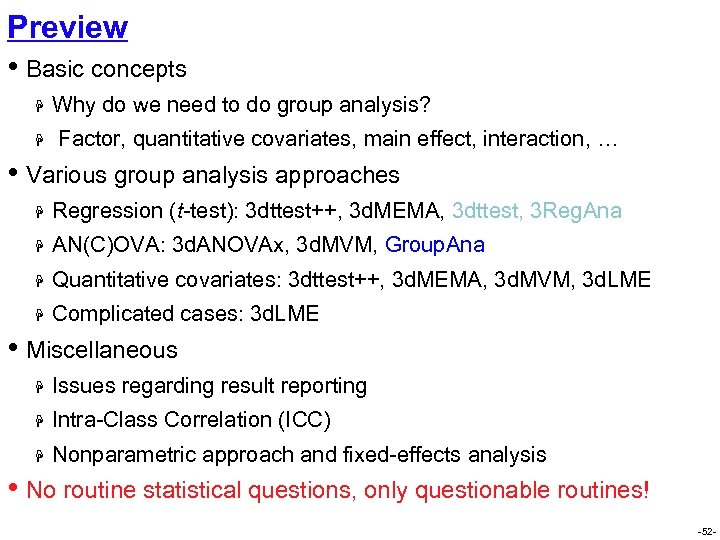Preview • Basic concepts H H Why do we need to do group analysis? Factor, quantitative covariates, main effect, interaction, … • Various group analysis approaches H Regression (t-test): 3 dttest++, 3 d. MEMA, 3 dttest, 3 Reg. Ana H AN(C)OVA: 3 d. ANOVAx, 3 d. MVM, Group. Ana H Quantitative covariates: 3 dttest++, 3 d. MEMA, 3 d. MVM, 3 d. LME H Complicated cases: 3 d. LME • Miscellaneous H Issues regarding result reporting H Intra-Class Correlation (ICC) H Nonparametric approach and fixed-effects analysis • No routine statistical questions, only questionable routines! -52 -